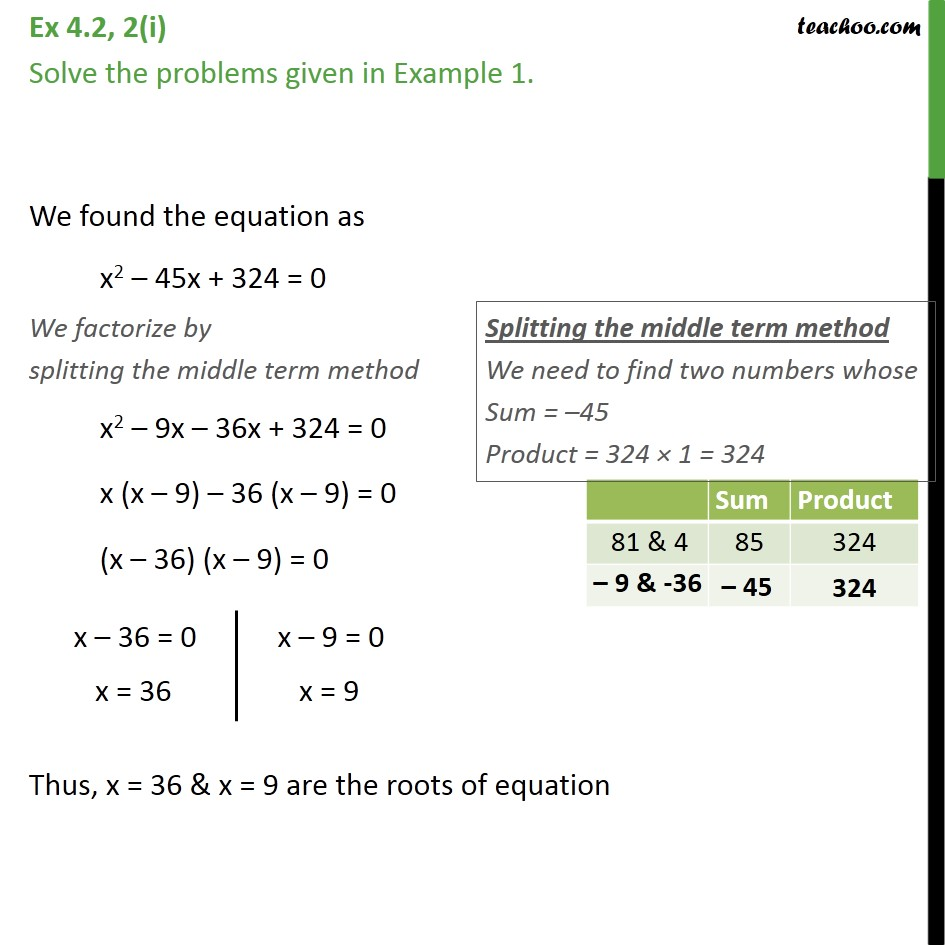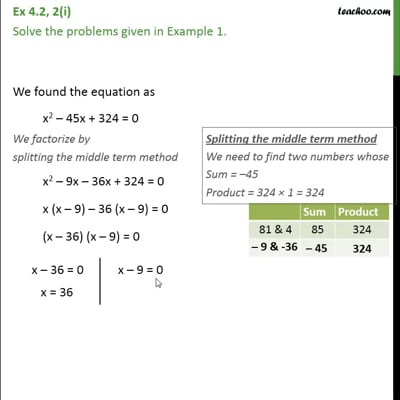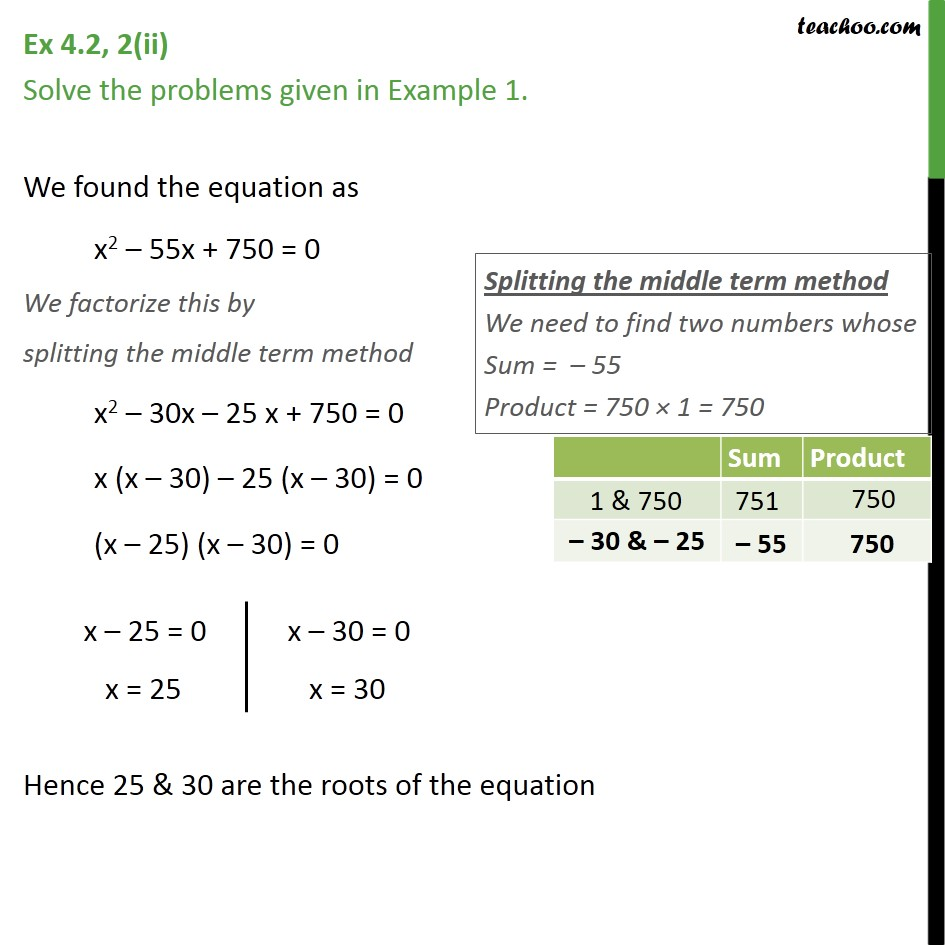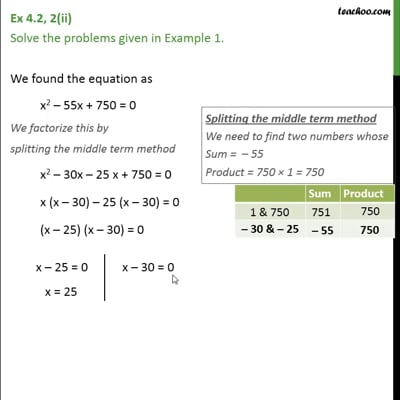Ex 4.2

Chapter 4 Class 10 Quadratic Equations (Term 2)
Serial order wiseThis video is only available for Teachoo black usersThis video is only available for Teachoo black users

### Transcript

Ex 4.2, 2(i) Solve the problems given in Example 1. We found the equation as x2 – 45x + 324 = 0 We factorize by splitting the middle term method x2 – 9x – 36x + 324 = 0 x (x – 9) – 36 (x – 9) = 0 (x – 36) (x – 9) = 0 Thus, x = 36 & x = 9 are the roots of equation Ex 4.2, 2(ii) Solve the problems given in Example 1. We found the equation as x2 – 55x + 750 = 0 We factorize this by splitting the middle term method x2 – 30x – 25 x + 750 = 0 x (x – 30) – 25 (x – 30) = 0 (x – 25) (x – 30) = 0 Hence 25 & 30 are the roots of the equation